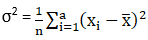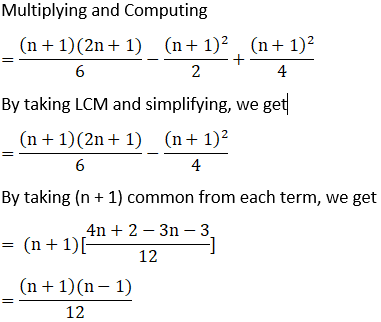Find the mean and variance for the first n natural numbers

Asked by Abhisek | 1 year ago |  76

##### Solution :-

We know that Mean =

$$\dfrac{Sum\; of\; all \;observations}{Number\; of \;observations}$$

Mean, x̅ = $$\dfrac{ ((n(n + 1))2)}{n}$$

$$\dfrac{ (n + 1)}{2}$$

and also Variance,By substitute that value of x̅ we get,We know that,

$$(a-b)^2=a^2-2ab+b^2$$(a + b)(a – b) = a2 – b2

σ2 = $$\dfrac{(n^2 – 1)}{12}$$

Mean = $$\dfrac{ (n + 1)}{2}$$ and Variance

$$\dfrac{(n^2 – 1)}{12}$$

Answered by Pragya Singh | 1 year ago

### Related Questions

#### Find the mean deviation from the mean and from a median of the following distribution

Find the mean deviation from the mean and from a median of the following distribution:

 Marks 0-10 10-20 20-30 30-40 40-50 No. of students 5 8 15 16 6

#### The age distribution of 100 life-insurance policy holders is as follows:

The age distribution of 100 life-insurance policy holders is as follows

 Age (on nearest birthday) 17-19.5 20-25.5 26-35.5 36-40.5 41-50.5 51-55.5 56-60.5 61-70.5 No. of persons 5 16 12 26 14 12 6 5

#### Compute mean deviation from mean of the following distribution:

Compute mean deviation from mean of the following distribution:

 Marks 10-20 20-30 30-40 40-50 50-60 60-70 70-80 80-90 No. of students 8 10 15 25 20 18 9 5

#### Find the mean deviation from the mean for the following data

Find the mean deviation from the mean for the following data:

 Classes 95-105 105-115 115-125 125-135 135-145 145-155 Frequencies 9 13 16 26 30 12